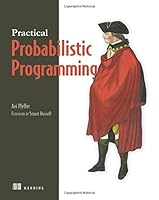Practical Probabilistic Programming0 Reviews
2016-05-01
325 pages

Book Description

Data accumulated about customers, products, and website users can not only help interpret the past, it can help predict the future! Probabilistic is a paradigm in which code models are used to draw probabilistic inferences from data. By applying specialized algorithms, programs assign degrees of probability to conclusions and make it possible to forecast future events like sales trends, system failures, experimental outcomes, and other critical concerns.

Practical Probabilistic Programming explains how to use the PP paradigm to model application domains and express those probabilistic models in code. It shows how to use the Figaro language to build a spam filter and apply Bayesian and Markov networks to diagnose computer system data problems and recover images. Then it dives into the world of probabilistic inference, where algorithms help turn the extended prediction of usage into a science. The book covers functional-style programming for text analysis and using object-oriented models to predict social phenomena like the spread of tweets, and using open universe models to model real-life usage. It also teaches the principles of algorithms such as belief propagation and Markov chain Monte Carlo. The book closes out with modeling dynamic systems by using a product cycle as its main example and explains how probabilistic models can help in the decision-making process for an ad campaign.

Purchase of the print book includes a free eBook in PDF, , and formats from Manning Publications.

Part 1 Introducing probabilistic programming and Figaro
Chapter 1 Probabilistic programming in a nutshell
Chapter 2 A quick Figaro tutorial
Chapter 3 Creating a probabilistic programming application

Part 2 Writing probabilistic programs
Chapter 4 Probabilistic models and probabilistic programs
Chapter 5 Modeling dependencies with Bayesian and Markov networks
Chapter 6 Using Scala and Figaro collections to build up models
Chapter 7 Object-oriented probabilistic modeling
Chapter 8 Modeling dynamic systems

Part 3 Inference
Chapter 9 The three rules of probabilistic inference
Chapter 10 Factored inference algorithms
Chapter 11 Sampling algorithms
Chapter 12 Solving other inference tasks
Chapter 13 Dynamic reasoning and parameter learning

Appendix A Obtaining and installing Scala and Figaro
Appendix B A brief survey of probabilistic programming systems

Book Details

• Title: Practical Probabilistic Programming
• Author:
• Length: 325 pages
• Edition: 1
• Language: English
• Publisher:
• Publication Date: 2016-05-01
• ISBN-10: 1617292338
• ISBN-13: 9781617292330